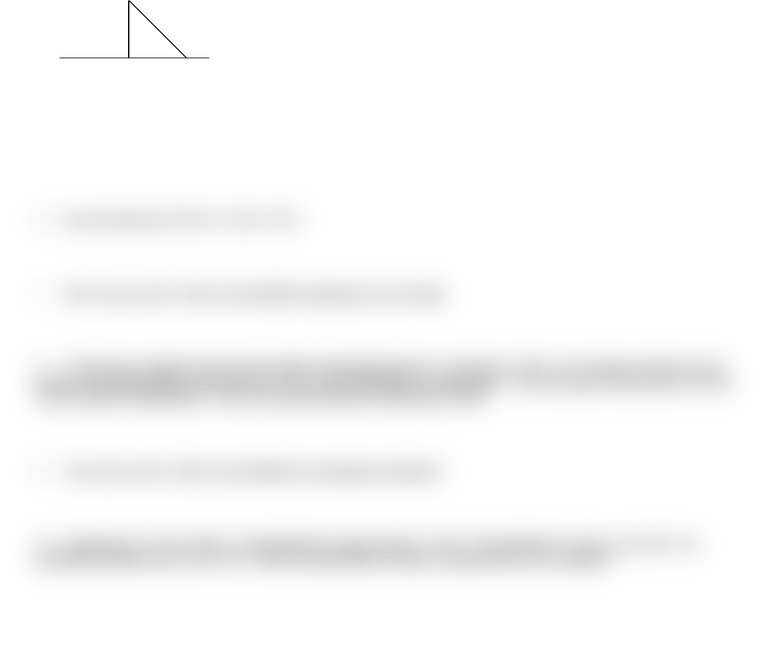Study Guides (380,000)
US (220,000)
UC-Irvine (3,000)
ECON (300)
Final

# ECON 15A Study Guide - Final Guide: Probability Density Function, Trail Of Broken Treaties, Poisson DistributionExam

Department
Economics
Course Code
ECON 15A
Professor
Paul Shirey
Study Guide
Final

This preview shows half of the first page. to view the full 2 pages of the document.NAME: _________________________________ I.D.: _____________________________________
FINAL
PROBABILITY AND STATISTICS, ECON 15A
DECEMBER 12, 2012
1. Lauren is typing up her dissertation. On average, she makes one typo for every two pages she types. What is the probability that she
will type one page and not make any typos? [Please use the Poisson distribution to answer this question.]
2 3. (Two questions twenty points) A jar contains eight ping-pong balls. Four are white; three are orange; and one is yellow.
Emily picks 960 ping-pong balls with replacement. What is the probability that between 369 and 381 balls are orange?
4. What is the probability density function (pdf) for the below graph? [I’m merely asking what f(x) is.]
0 1 2 3 4 5
5. Let X1 and X2 be two iid random variables. They each have a variance which we will call V(X). Explain why V(X1 + X2) = 2V(X)
while V(X1 + X1) = 4V(X). You need to explain how these cases differ.
6. Precisely when does V(X+Y) = V(X) + V(Y)?
7. Three cards are dealt. What is the probability of getting at least one King?
8. You left your car lights on for part of the night; the probability that your car will start is 79.6%. You also got a nail stuck in your
bike tire; the probability that it wont be flat is 25.7%. These probabilities are independent. You need to get to work and these are your
only two modes of transportation. What is the probability that you will not get to work?
9. Two cards are dealt. What is the probability of not getting any diamonds?
10. Gemma plans to sail to Catalina. The probability of seeing a porpoise is 30%. The probability of seeing a seal is 40%. The
probability of porpoise and a seal is 24%. What is the probability of seeing a seal given that she saw a porpoise?